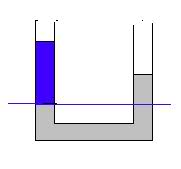# U-Shaped Tube Pressure Problem

## Homework Statement

A U shaped tube, open to the air on both ends, contains mercury. Water is poured into the left arm until the water column is 10 cm deep. How far upward from its intitial position does the mercury in the right arm rise?

## The Attempt at a Solution

h is the height of mercury on the left side of the tube
x is the distance that the mercury moves up

101300 Pa + (1000kg/m^3)(9.80)(.10m) + (13600kg/m^3)(9.80)h= 101300 Pa + (13,600kg/m^3)(9.80)(h+x)

980 + 133280h = 133280h + 133280x
980=133280x
x= .00735m = .735cm I'm not sure if I did this correctly--this answer seems quite small

alphysicist
Homework Helper
Hi bcjochim07,

It looks to me like you are answering the wrong question. In your equation x is the distance the top of the mercury is above the bottom of the water on the other side. However, they are asking for how much the mercury rose up above where it was before the water was poured in.

101300 Pa + (1000kg/m^3)(9.80)(.10m) = 101300 Pa + (13,600kg/m^3)(9.80)(x)

finally,

result: x/2

hint: you don't need to write the same pressure both side. draw a horizontal line from top of the lowest height side of most bottom liquid. And calculate the pressures for only the upper side of line which you draw.Last edited:
What did you mean by x/2? I got the same number for x using your equation that I did mine. I really didn't need to put the h in.

alphysicist
Homework Helper
x in your equation is the distance above the bottom of the water (where the purple line is in volcano's post). Pouring in the water pushes the mercury level down on the left side, and up on the right side. You found the difference in heights between the mercury levels on the left and right side; they want the difference in height between the new level on the right and the original level on the right.

If I try to visualize how it was before the water was added, I would just distributed the mercury evenly, so would I divide my answer by two then?

How much down(x/2), so much up(x/2) both sides. Think this, if one side down 10cm, how much rise other side? What is the distance difference between both sides?